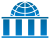# Bayesian statistics

Bayesian statistics, named for Thomas Bayes (1701–1761), is a theory in the field of statistics in which the evidence about the true state of the world is expressed in terms of degrees of belief known as Bayesian probabilities. Such an interpretation is only one of a number of interpretations of probability and there are other statistical techniques that are not based on 'degrees of belief'. One of the key ideas of Bayesian statistics is that "probability is orderly opinion, and that inference from data is nothing other than the revision of such opinion in the light of relevant new information."

## Outline

The general set of statistical techniques can be divided into a number of activities, many of which have special Bayesian versions.

### Statistical inference

Main article: Bayesian inference

Bayesian inference is an approach to statistical inference that is distinct from frequentist inference. It is specifically based on the use of Bayesian probabilities to summarize evidence.

### Statistical modeling

The formulation of statistical models using Bayesian statistics has the unique feature of requiring the specification of prior distributions for any unknown parameters. These prior distributions are as integral to a Bayesian approach to statistical modelling as the expression of probability distributions. Prior distributions can be either hyperparameters or hyperprior distributions.

### Design of experiments

The Bayesian design of experiments includes a concept called 'influence of prior beliefs'. This approach uses sequential analysis techniques to include the outcome of earlier experiments in the design of the next experiment. This is achieved by updating 'beliefs' through the use of prior and posterior distribution. This allows the design of experiments to make good use of resources of all types. An example of this is the multi-armed bandit problem.

### Statistical graphics

Statistical graphics includes methods for data exploration, for model validation, etc. The use of certain modern computational techniques for Bayesian inference, specifically the various types of Markov chain Monte Carlo techniques, have led to the need for checks, often made in graphical form, on the validity of such computations in expressing the required posterior distributions.

## References

1. Edwards, W.; Lindman, H.; Savage, L. J. (1963). "Bayesian Statistical Inference for Psycho-logical Research". Psychological Review. 70: 193–242. doi:10.1037/h0044139 (quote: pp 519-520). Cited as per Dennis Fryback's preface in O’Hagan, A.; Luce, B. (2003). "A primer on Bayesian Statistics in Health Economics and Outcomes Research" (PDF). Bayesian Initiative in Health Economics & Outcomes Research and the Centre for Bayesian Statistics in Health Economics. Retrieved June 9, 2015.Wikiversity has learning materials about Bayesian statistics